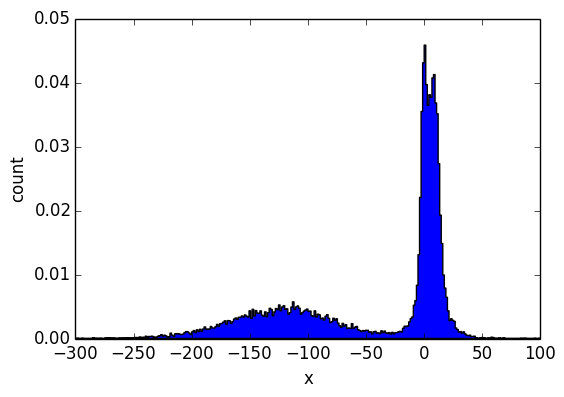This is the same stick breaking based mixture model found here: http://www.robots.ox.ac.uk/~fwood/anglican/examples/dp_mixture_model/index.html.

In :
using Stochy, Stochy.PyPlotSupport; import PyPlot

INFO: Loading help data...


The base distribution $H$ is a Normal-Gamma prior on the mean and variance of the observation distribution.

In :
@pp function H()
local var = 1. / ~Gamma(1, 0.1)
local mean = ~Normal(0, √(10var))
tuple(mean, √var)
end

Out:
H (generic function with 1 method)
In :
@pp repeat(H,10)

Out:
list((-13.010629950984848,3.3658996178312672), (-45.77174192030567,10.200900258898432), (15.659395597870311,5.997636239705596), (12.039330000234095,3.0488993843576835), (20.52601010262235,8.092445274356743), (-1.0594947705851219,2.0572991152389366), (3.418374191308808,4.3797571610579915), (-9.926381117382899,1.9772546235351978), (-3.8764835870871264,2.51166161352619), (-20.563800041458332,14.816846726072166))

params is a draw from a Dirichlet process with base distribution $H$. (This is often denoted $G$.)

In :
params = @pp DP(H, 1.72)

Out:
(anonymous function)

Making several draws from params shows the stochastic memoization effect. Note the repetition of some tuples below.

In :
@pp repeat(params, 10)

Out:
list((5.6686350451007,2.3294003390617277), (-7.383761228396905,8.57419987206087), (-18.77023112874221,3.8742570516808015), (-7.383761228396905,8.57419987206087), (-0.12056623523626799,2.062902797324893), (-18.77023112874221,3.8742570516808015), (-18.77023112874221,3.8742570516808015), (-7.383761228396905,8.57419987206087), (-18.77023112874221,3.8742570516808015), (-0.5958554634702551,3.853224991821724))

In the current implementation memoization is local to the calling @pp block. This means that the following is not equivilant to the cell above. Specifically, this performs one draw from each of 10 draws from the DP, whereas the code above makes 10 draws from a single DP.

In :
[@pp params() for _ in 1:10]

Out:
10-element Array{Any,1}:
(-4.936177893943471,2.5089731258345296)
(-2.048910317484265,2.319143625811254)
(-2.152587850602797,2.883942547573667)
(4.249136700418106,2.437442669739073)
(2.3501738100010194,2.34833772122104)
(8.319155325659535,4.9268395907623574)
(4.234836918935765,2.588754181982078)
(-4.404888763185945,5.874247584713328)
(102.52081478675677,21.85262939132541)
(-0.28919498802963073,1.5104630196787532)
In :
dist = @pp pmcmc(250,100) do
observe(Normal(params()...), 10)
observe(Normal(params()...), 11)
observe(Normal(params()...), 12)
observe(Normal(params()...), -100)
observe(Normal(params()...), -150)
observe(Normal(params()...), -200)
observe(Normal(params()...), 0.001)
observe(Normal(params()...), 0.01)
observe(Normal(params()...), 0.005)
observe(Normal(params()...), 0)
# Posterior predictive.
~Normal(params()...)
end;

In :
histogram(dist, bins=300, range=(-300,100), histtype="stepfilled");In [ ]: